## General Mechanical

•Gerald
Subscriber

Hi guys

I am trying to simulate a spinning(in the Z axis) slender piece of object with some ribs attached to it at about 1255.15k (982 degree C).

I was able to run and simulate everything correctly getting creep strain results of up to 1350 rad/s . However anything after that ansys would not run giving an error and warning. I would require it to be 2000rad/s.

The error :An unknown error occurred during solution. Check the Solver Output on the Solution Information object for possible causes.

warning: The unconverged solution (identified as Substep 999999) is output for analysis debug purposes. Results at this time should not be used for any other purpose.

I have uploaded the file onto my google drive link is blow.

Anyone knows how can i solve this problem?

•Sandeep Medikonda
Ansys Employee

Gerald,

Can you post snapshots of the force convergence plot, your model and any error messages seen in the solution output?

Regards,
Sandeep
Guidelines on the Student Community

•peteroznewman
Subscriber

Hi Gerald,

I opened your model and summarize below for Sandeep and other ANSYS members who are not permitted to open models.

You have a body that is 60 mm long, that starts 340 mm along the Y axis from the Global Csys.

The Thermal Condition is 982 C. Are you interested in simulating the thermal expansion from 22 C?
If not, you can just set the Environment Temperature to 982 C under Static Structural.
If you are, your material properties don't go down to 22 C and there is a warning about that.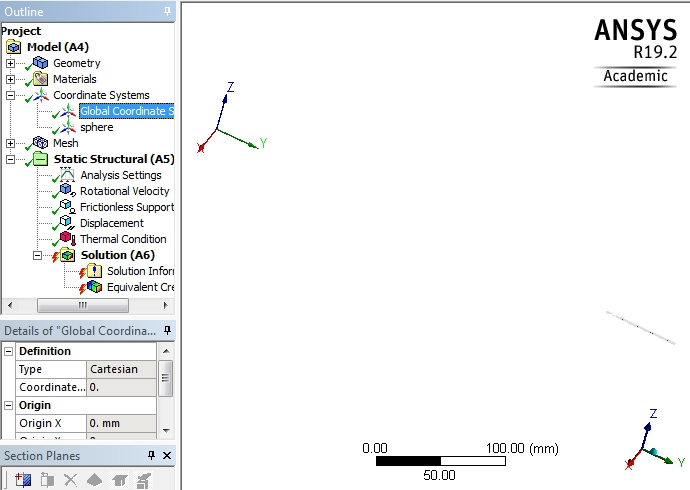You are spinning it around the Global Z axis at 2000 rad/s.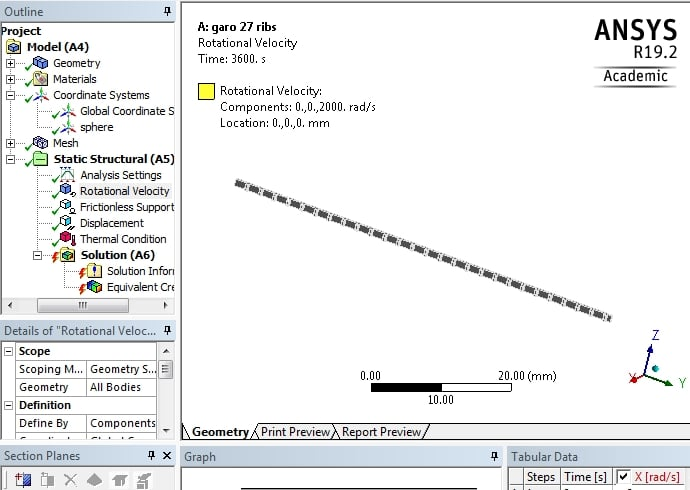You have Frictionless Support on two faces, the back side and the end face near the axis.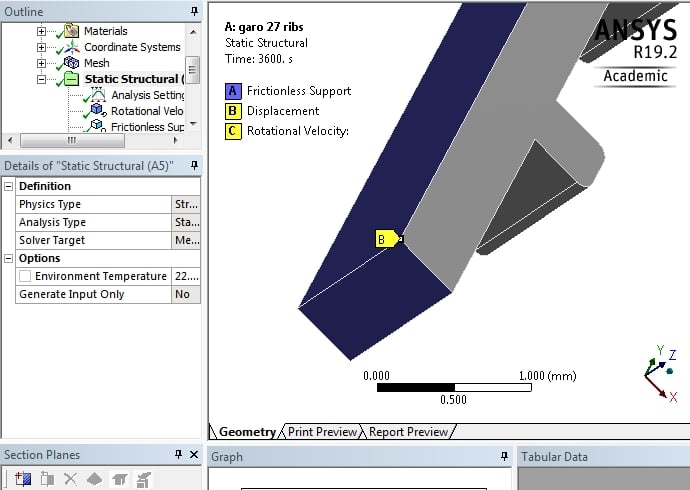You have a zero displacement on a Vertex. Best practice is to avoid applying BCs to a Vertex on solid elements.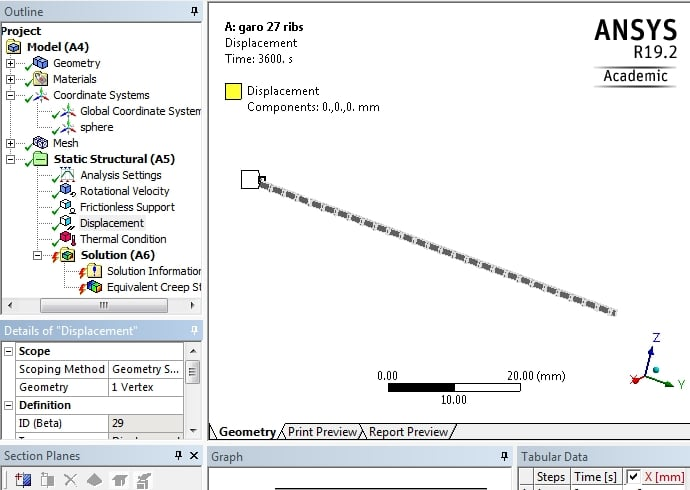Your Step Controls look like this: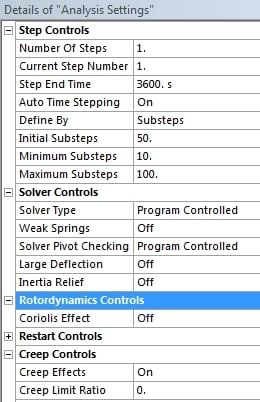The error when your model runs is this:

` *** WARNING ***                         CP =       2.044   TIME= 12 09 The creep integration algorithm does not converge for element 931,       material 1, creep model 8.                                              `

My suggestion: you should turn on Large Deflection under Analysis Settings. I'm not sure if you need Coriolis on or not.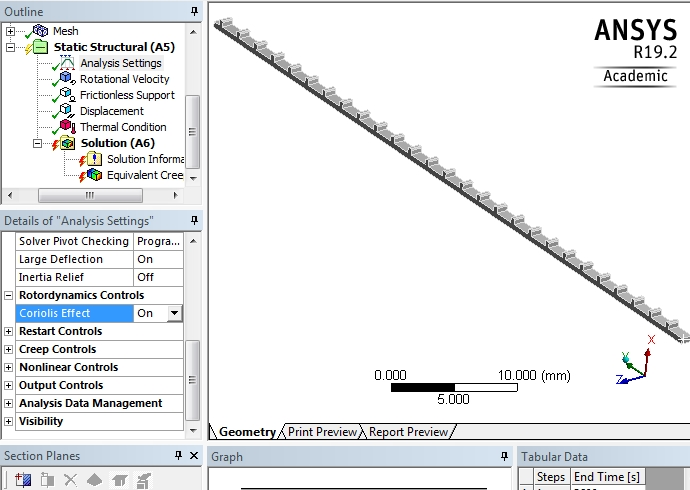I look forward to comments from other members.

Regards,
Peter

•Gerald
Subscriber

Hi Peter and Sandeep

Firstly thank you Peter for posting it up for me.

I have turned on both large deflection.

Edit: Once i turn on corollis but the error disappears and all results are 0.

May I know the reasoning why the BC with a hard vertex should be avoid?

Any other suggestion to aid the solving the error?

Reay appreciate the reply.

•Sandeep Medikonda
Ansys Employee

Gerald,

What does your material information specified in Engineering Data look like?

Also, can you post a snapshot of the force-convergence plot?

Regards,
Sandeep
Guidelines on the Student Community

•Gerald
Subscriber

Hi Sandeep,

Sorry for the late reply

For the material propery i have define a secondary creep with the constants shown below. (I have also attached the 2 images if better clarity is needed) sorry, i am not very good at this)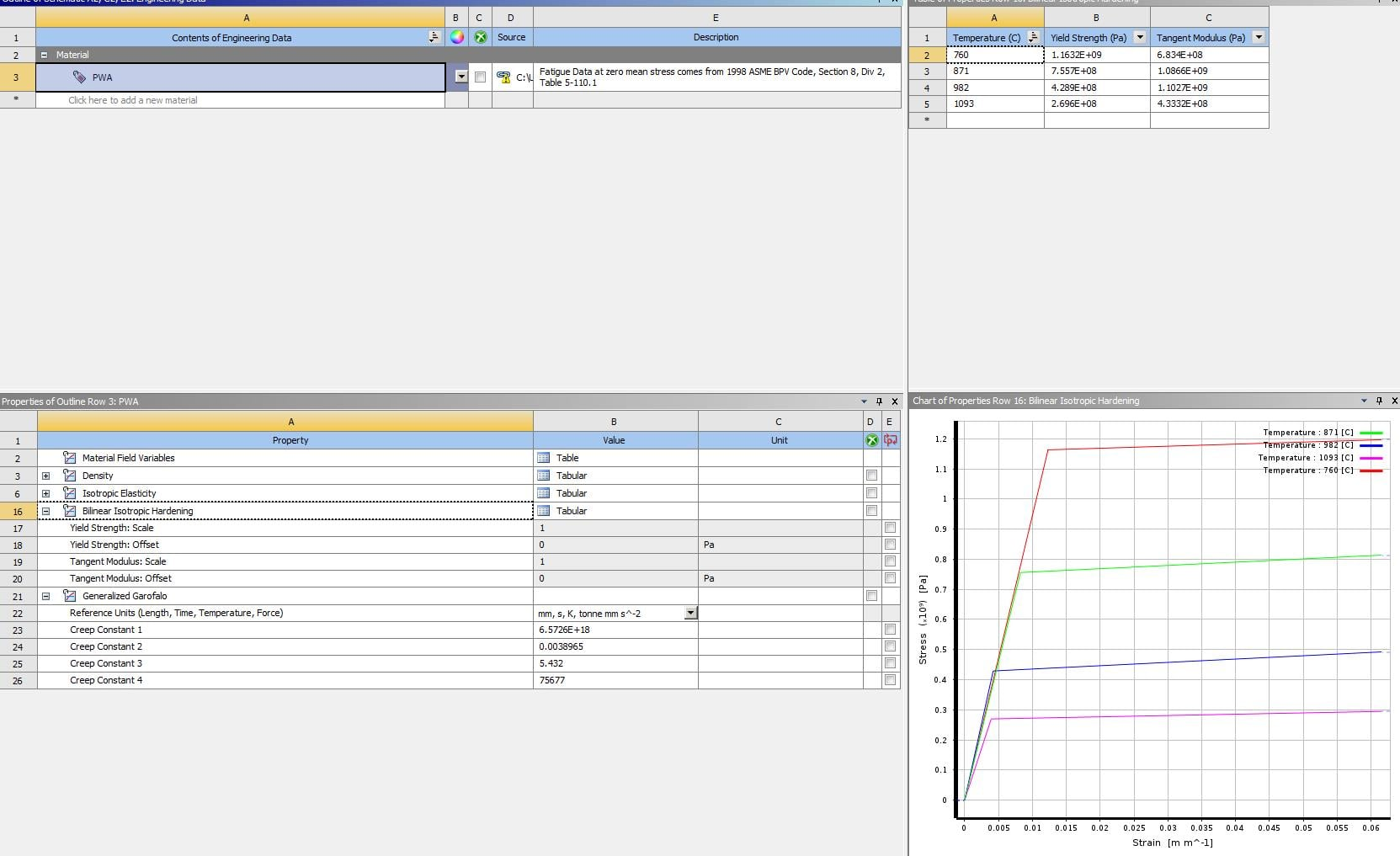And the force convergence plot (whch makes no sense to me)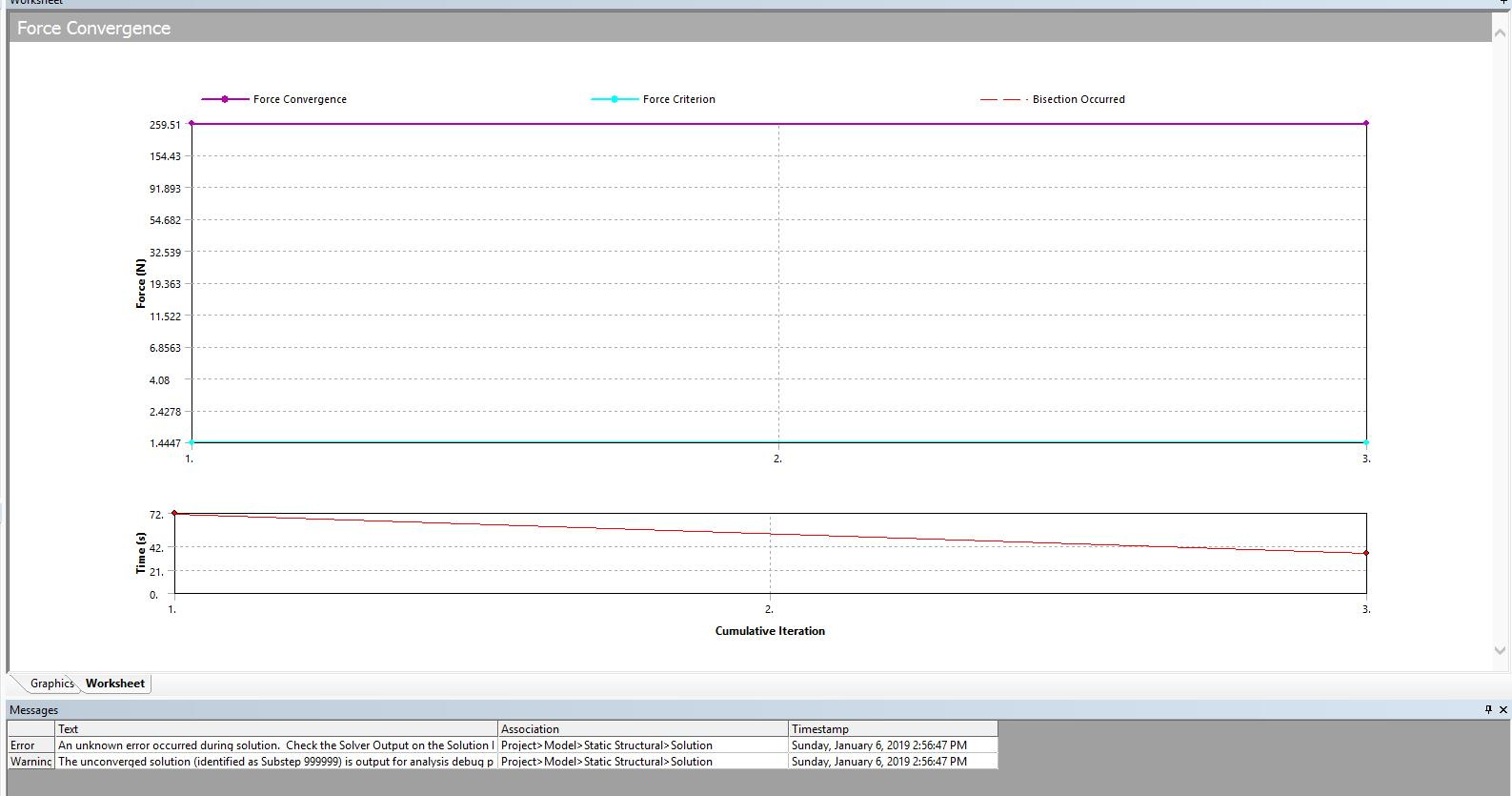•Sandeep Medikonda
Ansys Employee

Gerald,

Your simulation is failing to convergence at the very start itself. There might be something wrong in the material data inputs.

Can you go over this article and make sure you are following all the instructions suggested.

Also, what is the purpose of that displacement boundary condition? Are you trying to constrain rigid body motion? If so, constrain 3 different nodes to limit rigid body motion. Note that solid elements have only 3 degrees of freedom.

Lastly, try to separate your loads into different load steps.

Regards,
Sandeep

•peteroznewman
Subscriber

Gerald,

On the left is the tet mesh in your model that has mesh refinement at the bottom where the highest stress will be found, but it is too coarse for a good overall result. On the right is a hex mesh using the Sweep method of Mesh Control, but without the refinement at the bottom.

I'm currently running this mesh without creep, just to see the stress at 2000 radians/sec.

I wonder why you have a frictionless support on the back. This prevents the belt leaving that plane. Isn't it possible that the mass of the ribs will pull a section of belt between the ribs slightly away from the back? That would surely happen if the ribs were a bit taller. To see that effect, a contact must be used instead of a frictionless support. The contact can be frictionless, but that would allow the belt to lift slightly off the plane.

Ribbed belts like this are often engaged at both ends in some kind of wheel or clamp mechanism. This model has the near face constrained, but at the far end, there is no support along the belt axis. What is the real machine configuration at the far end? Isn't the ribbed belt engaged in something that would add a constraint at the far end too? If that is the case, the far end of the belt would go into compression and support some of the creep from occurring. It might also create slack in the belt that may allow the belt to buckle.

Regards,
Peter

•peteroznewman
Subscriber

I turned off creep in the Analysis settings to look at the stress in the part at 2000 radians/sec of rotational velocity. The model only reached 17 radians/sec before it encountered problems.

Here is the Force Convergence Plot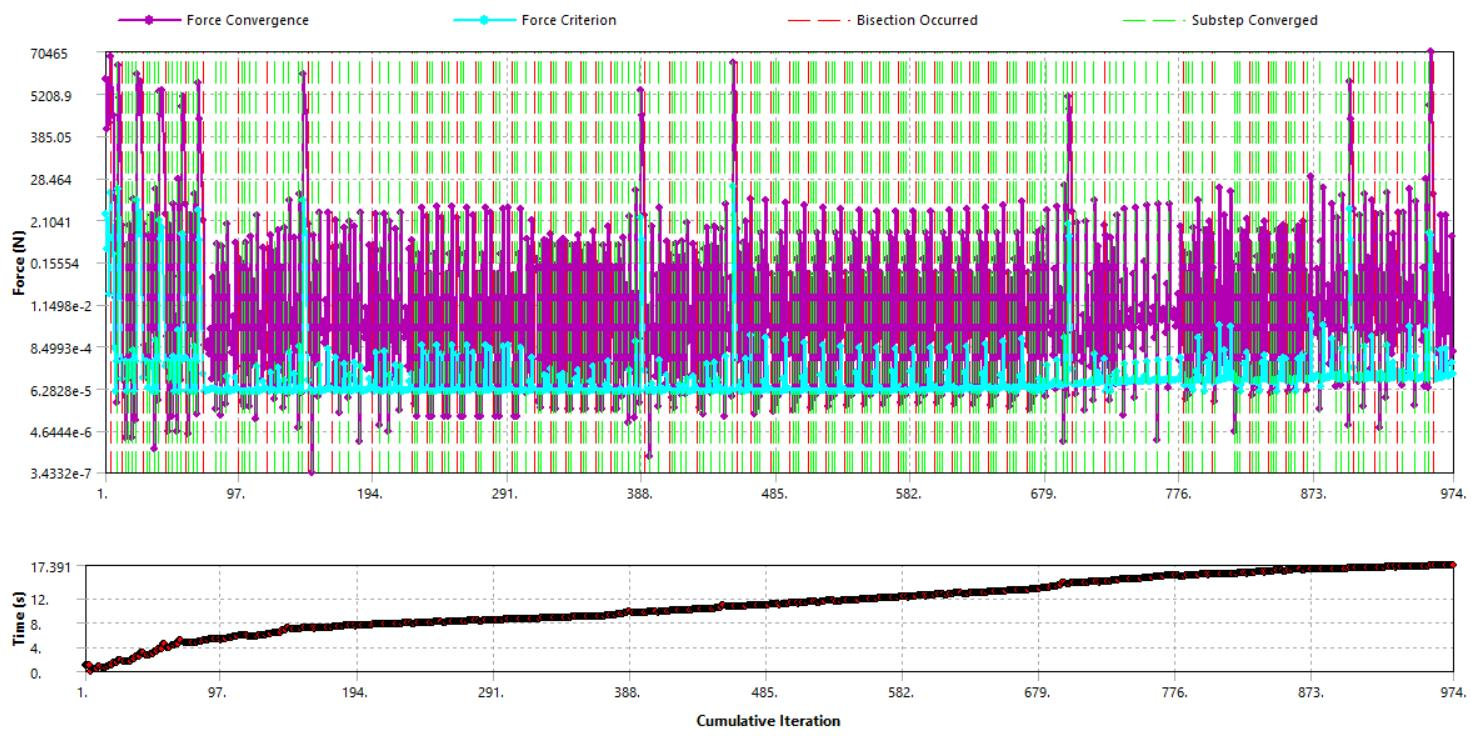Here is some of the output

```    >>> SOLUTION CONVERGED AFTER EQUILIBRIUM ITERATION   7
*** LOAD STEP     1   SUBSTEP   172  COMPLETED.    CUM ITER =    953
*** TIME =   17.2032         TIME INC =  0.500000E-01
*** AUTO TIME STEP:  NEXT TIME INC = 0.75000E-01  INCREASED (FACTOR = 1.5000)

FORCE CONVERGENCE VALUE  =   2664.      CRITERION=  0.4819
Writing NEWTON-RAPHSON residual forces to file: file.nr001
DISP CONVERGENCE VALUE   =  0.7100E-02  CRITERION=  0.7514E-02 <<< CONVERGED
EQUIL ITER   1 COMPLETED.  NEW TRIANG MATRIX.  MAX DOF INC= -0.7100E-02
FORCE CONVERGENCE VALUE  =   26.30      CRITERION=  0.2119E-02
Writing NEWTON-RAPHSON residual forces to file: file.nr002
DISP CONVERGENCE VALUE   =  0.3899E-02  CRITERION=  0.7667E-02 <<< CONVERGED
EQUIL ITER   2 COMPLETED.  NEW TRIANG MATRIX.  MAX DOF INC= -0.3899E-02
FORCE CONVERGENCE VALUE  =  0.5999E-01  CRITERION=  0.1253E-03
Writing NEWTON-RAPHSON residual forces to file: file.nr003
DISP CONVERGENCE VALUE   =  0.2028E-04  CRITERION=  0.7824E-02 <<< CONVERGED
EQUIL ITER   3 COMPLETED.  NEW TRIANG MATRIX.  MAX DOF INC=  0.2028E-04
FORCE CONVERGENCE VALUE  =  0.6560E-04  CRITERION=  0.1168E-03 <<< CONVERGED
Writing NEWTON-RAPHSON residual forces to file: file.nr004
>>> SOLUTION CONVERGED AFTER EQUILIBRIUM ITERATION   3
*** LOAD STEP     1   SUBSTEP   173  COMPLETED.    CUM ITER =    956
*** TIME =   17.2782         TIME INC =  0.750000E-01
*** AUTO TIME STEP:  NEXT TIME INC = 0.11250      INCREASED (FACTOR = 1.5000)

FORCE CONVERGENCE VALUE  =   5741.      CRITERION=   1.219
Writing NEWTON-RAPHSON residual forces to file: file.nr005
DISP CONVERGENCE VALUE   =   8.072      CRITERION=  0.4118
EQUIL ITER   1 COMPLETED.  NEW TRIANG MATRIX.  MAX DOF INC=  -8.072
FORCE CONVERGENCE VALUE  =   2448.      CRITERION=  0.9374
Writing NEWTON-RAPHSON residual forces to file: file.nr006
DISP CONVERGENCE VALUE   =   8.179      CRITERION=  0.4203
EQUIL ITER   2 COMPLETED.  NEW TRIANG MATRIX.  MAX DOF INC=   8.179
FORCE CONVERGENCE VALUE  =  0.7047E+05  CRITERION=  0.8472
Writing NEWTON-RAPHSON residual forces to file: file.nr001
DISP CONVERGENCE VALUE   =   53.60      CRITERION=   2.853
EQUIL ITER   3 COMPLETED.  NEW TRIANG MATRIX.  MAX DOF INC=   53.60

*** ERROR ***                           CP =    2192.062   TIME= 16:47:02
Element 12841 (SOLID185) is turning inside out.

*** ERROR ***                           CP =    2192.062   TIME= 16:47:02
Element 1769 (SOLID185) is turning inside out.

*** ERROR ***                           CP =    2192.062   TIME= 16:47:02
Element 18145 (SOLID185) is turning inside out.
*** LOAD STEP     1   SUBSTEP   174  NOT COMPLETED.  CUM ITER =    960
*** BEGIN BISECTION NUMBER   1    NEW TIME INCREMENT=  0.50000E-01

FORCE CONVERGENCE VALUE  =   1756.      CRITERION=  0.3173
Writing NEWTON-RAPHSON residual forces to file: file.nr002
DISP CONVERGENCE VALUE   =  0.4793E-02  CRITERION=  0.7514E-02 <<< CONVERGED
EQUIL ITER   1 COMPLETED.  NEW TRIANG MATRIX.  MAX DOF INC= -0.4793E-02
FORCE CONVERGENCE VALUE  =   10.79      CRITERION=  0.7491E-03
Writing NEWTON-RAPHSON residual forces to file: file.nr003
DISP CONVERGENCE VALUE   =  0.1690E-02  CRITERION=  0.7667E-02 <<< CONVERGED
EQUIL ITER   2 COMPLETED.  NEW TRIANG MATRIX.  MAX DOF INC= -0.1690E-02
FORCE CONVERGENCE VALUE  =  0.1106E-01  CRITERION=  0.1166E-03
Writing NEWTON-RAPHSON residual forces to file: file.nr004
DISP CONVERGENCE VALUE   =  0.6397E-04  CRITERION=  0.7824E-02 <<< CONVERGED
EQUIL ITER   3 COMPLETED.  NEW TRIANG MATRIX.  MAX DOF INC= -0.6397E-04
FORCE CONVERGENCE VALUE  =  0.5714E-03  CRITERION=  0.1170E-03
Writing NEWTON-RAPHSON residual forces to file: file.nr005
DISP CONVERGENCE VALUE   =  0.1221E-02  CRITERION=  0.7983E-02 <<< CONVERGED
EQUIL ITER   4 COMPLETED.  NEW TRIANG MATRIX.  MAX DOF INC= -0.1221E-02
FORCE CONVERGENCE VALUE  =  0.2141      CRITERION=  0.1588E-03
Writing NEWTON-RAPHSON residual forces to file: file.nr006
DISP CONVERGENCE VALUE   =  0.3526E-05  CRITERION=  0.8146E-02 <<< CONVERGED
EQUIL ITER   5 COMPLETED.  NEW TRIANG MATRIX.  MAX DOF INC= -0.3526E-05
FORCE CONVERGENCE VALUE  =  0.1619E-03  CRITERION=  0.1217E-03
Writing NEWTON-RAPHSON residual forces to file: file.nr001
DISP CONVERGENCE VALUE   =  0.4386E-02  CRITERION=  0.8313E-02 <<< CONVERGED
EQUIL ITER   6 COMPLETED.  NEW TRIANG MATRIX.  MAX DOF INC=  0.4386E-02
FORCE CONVERGENCE VALUE  =   2.760      CRITERION=  0.6774E-03
Writing NEWTON-RAPHSON residual forces to file: file.nr002
DISP CONVERGENCE VALUE   =  0.7357E-05  CRITERION=  0.8482E-02 <<< CONVERGED
EQUIL ITER   7 COMPLETED.  NEW TRIANG MATRIX.  MAX DOF INC= -0.7357E-05
FORCE CONVERGENCE VALUE  =  0.1875E-02  CRITERION=  0.1264E-03
Writing NEWTON-RAPHSON residual forces to file: file.nr003
DISP CONVERGENCE VALUE   =  0.3783E-03  CRITERION=  0.8655E-02 <<< CONVERGED
EQUIL ITER   8 COMPLETED.  NEW TRIANG MATRIX.  MAX DOF INC=  0.3783E-03
FORCE CONVERGENCE VALUE  =  0.2060E-01  CRITERION=  0.1334E-03
Writing NEWTON-RAPHSON residual forces to file: file.nr004
DISP CONVERGENCE VALUE   =  0.3448E-04  CRITERION=  0.8832E-02 <<< CONVERGED
EQUIL ITER   9 COMPLETED.  NEW TRIANG MATRIX.  MAX DOF INC= -0.3448E-04
FORCE CONVERGENCE VALUE  =  0.1572E-03  CRITERION=  0.1320E-03
Writing NEWTON-RAPHSON residual forces to file: file.nr005
DISP CONVERGENCE VALUE   =  0.4438E-02  CRITERION=  0.9012E-02 <<< CONVERGED
EQUIL ITER  10 COMPLETED.  NEW TRIANG MATRIX.  MAX DOF INC= -0.4438E-02
FORCE CONVERGENCE VALUE  =   2.836      CRITERION=  0.7511E-03
Writing NEWTON-RAPHSON residual forces to file: file.nr006
DISP CONVERGENCE VALUE   =  0.7579E-05  CRITERION=  0.9196E-02 <<< CONVERGED
EQUIL ITER  11 COMPLETED.  NEW TRIANG MATRIX.  MAX DOF INC= -0.7579E-05
FORCE CONVERGENCE VALUE  =  0.2169E-02  CRITERION=  0.1370E-03
Writing NEWTON-RAPHSON residual forces to file: file.nr001
DISP CONVERGENCE VALUE   =  0.3224E-03  CRITERION=  0.9384E-02 <<< CONVERGED
EQUIL ITER  12 COMPLETED.  NEW TRIANG MATRIX.  MAX DOF INC=  0.3224E-03
FORCE CONVERGENCE VALUE  =  0.1491E-01  CRITERION=  0.1434E-03
Writing NEWTON-RAPHSON residual forces to file: file.nr002
DISP CONVERGENCE VALUE   =  0.4691E-04  CRITERION=  0.9575E-02 <<< CONVERGED
EQUIL ITER  13 COMPLETED.  NEW TRIANG MATRIX.  MAX DOF INC= -0.4691E-04
FORCE CONVERGENCE VALUE  =  0.3045E-03  CRITERION=  0.1431E-03
Writing NEWTON-RAPHSON residual forces to file: file.nr003
DISP CONVERGENCE VALUE   =  0.2277E-02  CRITERION=  0.9771E-02 <<< CONVERGED
EQUIL ITER  14 COMPLETED.  NEW TRIANG MATRIX.  MAX DOF INC= -0.2277E-02
FORCE CONVERGENCE VALUE  =  0.7441      CRITERION=  0.3177E-03
Writing NEWTON-RAPHSON residual forces to file: file.nr004
DISP CONVERGENCE VALUE   =  0.1855E-05  CRITERION=  0.9970E-02 <<< CONVERGED
EQUIL ITER  15 COMPLETED.  NEW TRIANG MATRIX.  MAX DOF INC= -0.1855E-05
FORCE CONVERGENCE VALUE  =  0.5967E-03  CRITERION=  0.1488E-03
Writing NEWTON-RAPHSON residual forces to file: file.nr005

*** ERROR ***                           CP =    2220.406   TIME= 16:471
Solution not converged at time 17.3282473 (load step 1 substep 174).
Run terminated.

*** WARNING ***                         CP =    2220.406   TIME= 16:471
The unconverged solution (identified as time 100 substep 999999) is
output for analysis debug purposes.  Results should not be used for
any other purpose.   ```

The solver just gave up trying to converge.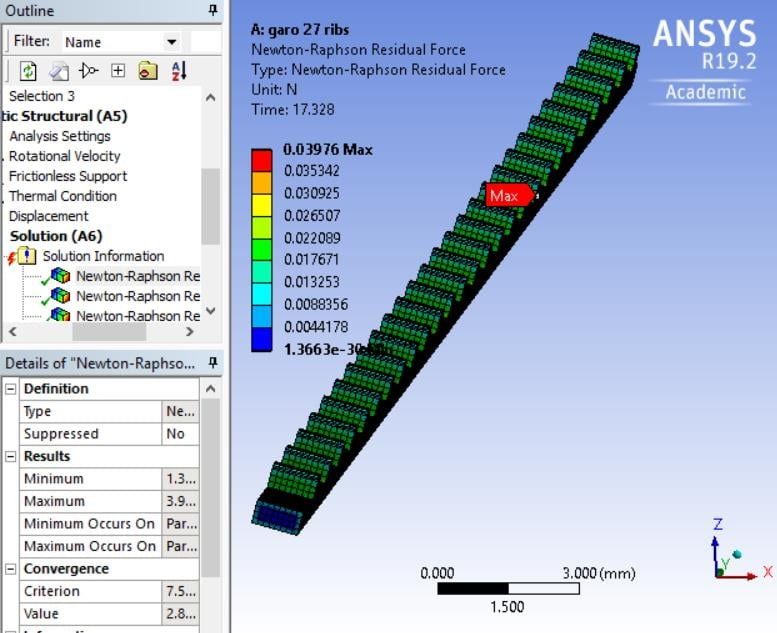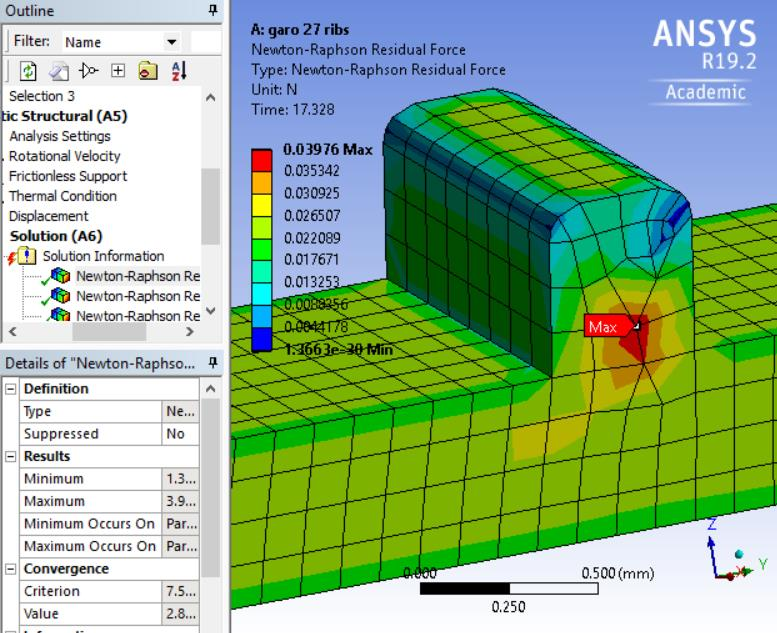I'm interested to hear some input on this model.

Regards,
Petet

•jj77
Subscriber

Why it does not converge (without creep), is because at ~ 980 celcius the whole fixed cross section yields, thus there is no way of balancing the centrifugal loads. At 760 say, it is OK, since the part there has not yielded yet. At 960 yielding occurs at half the value of 760 celcius (~1100 MPa). At 760 it is also ok with creep, but units creep constants (mm,..) need to be the same of course as in mechanical workbench (mm,..).

I should also point out like mentioned this body is sweepable, thus a structured HEX mesh was used (elements size of about 0.2 mm)

•peteroznewman
Subscriber

jj77, the yield strength for the material at 982 C is 428.9 MPa.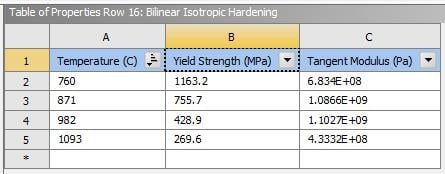The maximum equivalent stress in the part at 34 radians/sec is 0.55 MPa, so I don't think the convergence failure is due to yield across the whole cross section. [EDIT: Step 1 takes 100 seconds and goes to 200 radians/sec so 17 seconds is 34 radians/sec]

I think it just needs smaller elements to converge, but I am bumping into the student limit here. I could make the elements twice as wide while making them smaller in the other directions to see if that makes the model get further along, but I am more interested to read an answer from Gerald about how the belt is supported in the real machine and whether the constraints in the model are a good representation of the real machine.

Regards,
Peter

•jj77
Subscriber
Peteroznewman do not what you do but the rotation is 2000 rad sec as it is in the model, not sure where you get 17 from. so trust me it fails due to plastic hinge on the entire fixed cross section as I said loads cannot be balanced by the reaction force at the fixed support. (Just run with only large deflections no material nl, and one can see that vm is way above yield). That is why it could be solved for 1300 rad/sec and not 2000, since the later is creating the full plastic section.
•peteroznewman
Subscriber

jj77, I expect the solver may fail to converge before reaching 2000 rad/sec due to a plastic hinge. The model I ran above ramps the rotational velocity from 0 to 200 rad/sec in 100 seconds in step 1, so at 17 seconds when the solver fails to converge, the rotational velocity is only 34 radians/sec (I edited the post above when I realized I didn't use 100 rad/sec at 100 seconds as I had assumed). At that time, the material is still in the elastic range according to the stress result so there is zero plastic strain. That's the same as having no material nl.

I changed the mesh to have six elements across the belt and rib where before I had only four elements. The solver is running now, but has already gone further than it did before. That is what I mean about convergence in that model needing smaller elements.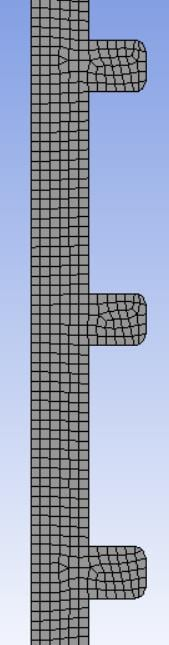The motivation to get a uniform mesh along the length was because the tet mesh was too coarse to compute an accurate creep strain because the large elements did not capture the variation in stress over the cross section and since the creep rate is a function of stress, a significant error in creep strain could occur.

Regards,
Peter

•Gerald
Subscriber

Hi peter,

First of all sorry for the delayed reply was not able to get online till now.

This is not really a machine. The idea of simulating this is to see creep in rib tabulators used in turbine blades. Therefore, the constrains are only applied at an single end (which is the roots 2 frictionless support and a 0 displacement at the corner) and rotating in Z.

I have managed to push the rotation velocity to to 1400 Rad/s by decreasing the time step setting to all 1 (minimum and maximum time step) with similar mesh sizes as you did.

However i realize the more refined it gets the lesser Rad/s it  can accepts.(i am not sure why though) it may have to do with accuracy of the results.

Hope this helps.

Anyways to all,

I appreciate all the replies.

•peteroznewman
Subscriber

Hi Gerald,

Do you mean rib turbulators? Please explain more about what they are and how they are constrained. You can call it a structure if you like, but that part is connected to the rest of the structure in some way. Where and how is it connected?

As jj77 has pointed out, the material near the inner supported face goes fully plastic around 1460 radians/sec rotational velocity in this model, so there is no equilibrium beyond that. That is the failure velocity for the temperature, materials and geometry you have specified.

Very coarse elements can't properly calculate the stress so they miss the failure behavior that the small elements will exhibit.

Regards,
Peter

•Gerald
Subscriber

Hi peter,

These rib turbulator are found inside a turbine blade and it is spinning. A picture is shown below.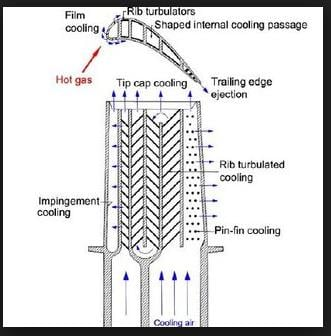I am modelling just the center portion of it to analyse where is highest creep point would occur. this whole blade is then connected to a rotating disk.

With regards to the temperature and failure, i understand the higher the temperature the lower the yield strength(fail at lower stress) this was pointed out by you in one of the previous post. what if i do a temperature gradient i.e lower temperature at the base higher in the center as shown in the picture below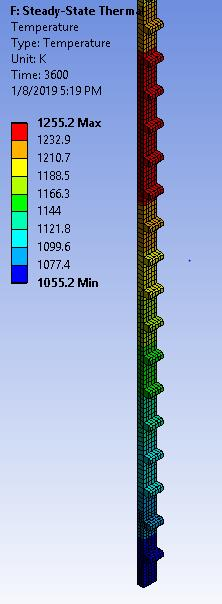and i run it at 1900 Rad/s the results is as shown in terms of equivalent stress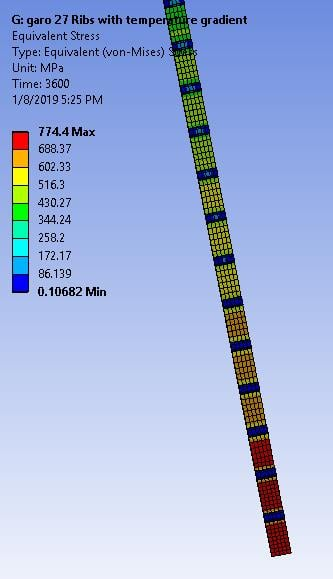Would it be possible to know which part of it is closest to yield/failing because different portion has a different temperature thus different yield strength.

Regards,

Gerald

•peteroznewman
Subscriber

Gerald,

Thanks for the illustration that shows a rib turbulator in a cooling cavity of a turbine blade that is spinning.

I want to see more detail on the assembly of the rib turbulator into the cooling cavity of the turbine blade.

The rib turbulator is not loosely hanging in the turbine cooling cavity. Isn't it bonded to the wall of the cavity?

If it is bonded to the wall of the cavity, then the frictionless support you show is not a good representation of the way the rib turbulator is supported by the turbine cavity wall.

I think you have to get the connection to the turbine cavity wall correct before you work on creep at some temperature.

Regards,
Peter